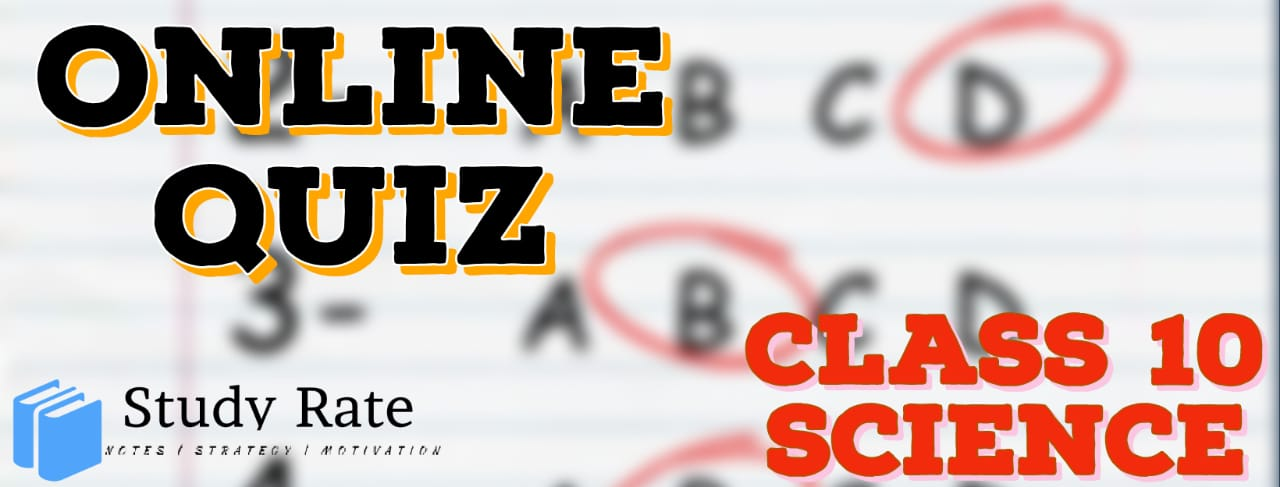## CBSE Class 10 Science Light – Reflection & Refraction MCQ Quiz with Answers

Free PDF Download of CBSE Class 10 Science Chapter 10 Light – Reflection & Refraction Multiple Choice Questions with Answers. MCQ Questions for Class 10 Science with Answers were Prepared Based on Latest Exam Pattern. Students can solve NCERT Class 10 Science Light – Reflection & Refraction Multiple Choice Questions with Answers to know their preparation level.

Light – Reflection & Refraction Class 10 Science MCQ Quiz Online

1. An object is placed 20 cm in front of a plane mirror. The mirror is moved 2 cm towards the object. The distance between the positions of the original and final images seen in the mirror is:

2.  A ray of light that strikes a plane mirror PQ at an angle of incidence of 30o, is reflected from the plane mirror and then strikes a second plane mirror QR placed at right angles to the first mirror. The angle of reflection at the second mirror is:

3. An object is placed at 100 mm in front of a concave mirror which produces an upright image (erect image). The radius of curvature of the mirror is:

4. Which position of the object will produce a magnified virtual image, if a concave mirror of focal length 15 cm is being used?

5. A concave mirror produces a magnification of +4. The object is placed:

6. Two big mirrors A and B are fitted side by side on a wall. A man is standing at such a distance from the wall that he can see the erect image of his face in both the mirrors. When the man starts walking towards the mirrors, he finds that the size of his face in mirror A goes on increasing but that in mirror B remains the same:

7. A ray of light is travelling in a direction perpendicular to the boundary of a parallel glass slab. The ray of light:

8. A lens of focal length 12 cm forms an erect image, three times the size of the object. The distance between the object and image is:

9. If an object is placed 21 cm from a converging lens, the image formed is slightly
smaller than the object. If the object is placed at a distance of 19 cm from the lens,
the image formed is slightly larger than the object. The approximate focal length of
the lens is:

10.  A student does the experiment on tracing the path of a ray of light passing through a rectangular glass slab for different angles of incidence. He can get a correct measure of the angle of incidence and the angle of emergence by following the labelling indicated in figure:11.  A student studies that a convex lens always forms a virtual image irrespective of its position. What causes the convex mirror to always form a virtual image?

12. Rahul conducts an experiment using an object of height 10 cm and a concave lens with focal length 20 cm. The object is placed at a distance of 25 cm from the lens. Can the image be formed on a screen?

13. An object is placed at a distance of 40cm in front of a concave mirror of focal length 20cm.The image produced is:

14. Magnification produced by a rear view mirror fitted in vehicles

15. You are given water, mustard oil, glycerine and kerosene. In which of these media a ray of light incident obliquely at same angle would bend the most?

16. The mirror having reflecting surface curved inwards

17. A 10 mm long awl pin is placed vertically in front of a concave mirror. A 5 mm long image of the awl pin is formed at 30 cm in front of the mirror. The focal length of this mirror is

18.
Badal Appeared In Class Test But He Confused To Know The Correct Statement. Would You Help Him To Know That?

19. These Days New Cartesian Sign Convention Is Used For Measuring The Various Distance In The Ray Diagrams Of Spherical Mirror. Choose The Correct Statements:

20.
In A Science Quiz Competition, Ritika Are Asked A Question Where She Had To Choose The Statement Which Was/Were Incorrect?